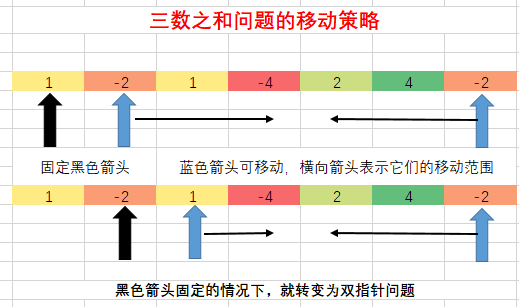zoukankan      html  css  js  c++  java

# LeetCode：最接近的三数之和【16】

### 题目描述

给定一个包括 n 个整数的数组 `nums` 和 一个目标值 `target`。找出 `nums` 中的三个整数，使得它们的和与 `target` 最接近。返回这三个数的和。假定每组输入只存在唯一答案。

```例如，给定数组 nums = [-1，2，1，-4], 和 target = 1.

与 target 最接近的三个数的和为 2. (-1 + 2 + 1 = 2).
```

### 题目分析

这道题就是三数之和问题的一种变形

三数之和问题的求解策略是将三指针变为双指针问题，即固定一个指针（黑色）。每一轮移动两个蓝色指针中，不断刷新结果值### Java题解

```class Solution {
public int threeSumClosest(int[] nums, int target) {
//假设某种可能情况，很大程度上不是最优的
int res = nums+nums+nums[nums.length-1];
Arrays.sort(nums);
for(int i=0;i<nums.length-2;i++)
{
int start = i+1;
int end = nums.length-1;
while(start<end)
{
int sum = nums[i]+nums[start]+nums[end];
if(sum>target)
end--;
if(sum<=target)
start++;
if(Math.abs(sum-target)<Math.abs(res-target))
res=sum;
}
}
return res;
}
}
```
• 相关阅读:
使用node 创建一个新项目
安装node 及相关配置
Java面试查漏补缺
科学计算软件——Octave安装
Wine——在Linux上运行Windows软件
windows和linux中搭建python集成开发环境IDE——如何设置多个python环境
Ubuntu安装Gnome3
ubuntu自定义分辨率
Ubuntu添加开机自动启动程序方法
sublime Text3及其插件的使用
• 原文地址：https://www.cnblogs.com/MrSaver/p/9497363.html